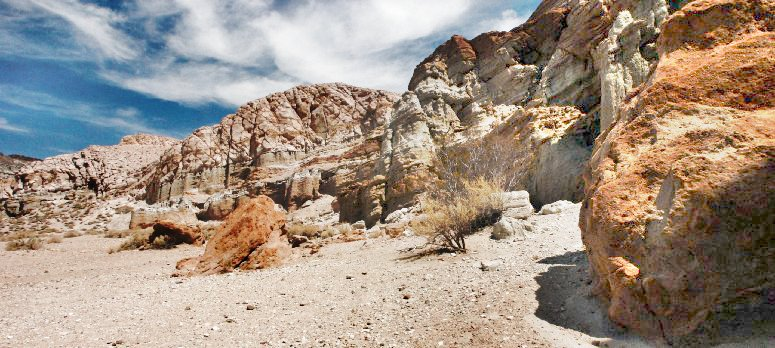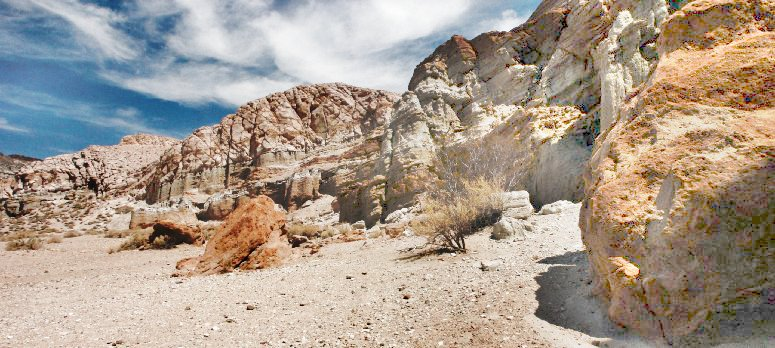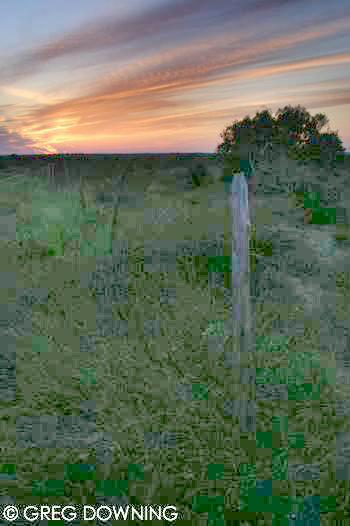## DUALTONEMAP

 Enhances the shadows and/or highlight regions in an image.

### EXAMPLES

 Example 1 Original -m shadows -s 0,30 -m shadows -s 0,100 -cExample 2 OriginalArguments: -m shadows -s 0,40 Arguments: -m shadows -s 0,100 -cExample 3 OriginalArguments: -m shadows -s 0,30 Arguments: -m shadows -s 0,100,0.9 -cExample 4 OriginalArguments: -m shadows -s 0,30 Arguments: -m shadows -s 0,80 -cExample 5 OriginalArguments: -m both -s 0,30,2 -h 20,100Arguments: -m both -s 0,70 -h 50,100 -cArguments: -m both -s 0,70,1.5 -h 50,100 -cExample 6 OriginalArguments: -m shadows -s 0,8 Arguments: -m both -s 0,45 -h 50,100 -cExample 7 Original (Actual HDR Format Used)Arguments: -m both -s 0,18 -h 18,100 -c -r 3Example 8 Original (Actual HDR Format Used)Arguments: -m shadows -s 0,25 -c -r 3Arguments: -m shadows -s 0,30 -c -r 3 -t25What the script does is as follows: Computes the mean of the image Thresholds the image at the mean Blurs the thresholded image over the ramp distance Applies the highlight -level parameters to the input image Applies the shadow -level parameters to the input image Composites the two modified images using the mask image to generate the output This is equivalent to the following IM commands if [ "\$cmode" = "yes" ]; then colormode="-set colorspace sRGB -colorspace RGB" else colormode="" fi convert -quiet -regard-warnings "\$infile" \$colormode +repage "\$tmpA1" thresh=`convert \$tmpA1 -format "%[fx:100*(mean+\$bias/100)]" info:` convert \$tmpA1 -threshold \${thresh}% -blur \${ramp}x65000 \$tmpA2 if [ "\$mode" = "both" ]; then convert \$tmpA1 -level \${sbp},\${swp},\${sg}% \$tmpA3 convert \$tmpA1 -level \${hbp},\${hwp},\${hg}% \$tmpA4 convert \$tmpA3 \$tmpA4 \$tmpA2 -compose over -composite \$outfile elif [ "\$mode" = "shadows" ]; then convert \$tmpA1 -level \${sbp},\${swp},\${sg}% \$tmpA3 convert \$tmpA3 \$tmpA1 \$tmpA2 -compose over -composite \$outfile elif [ "\$mode" = "highlights" ]; then convert \$tmpA1 -level \${hbp},\${hwp},\${hg}% \$tmpA4 convert \$tmpA1 \$tmpA4 \$tmpA2 -compose over -composite \$outfile fi